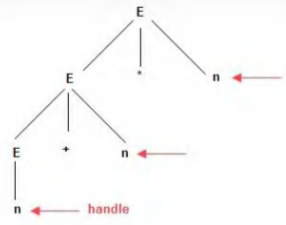##### For grammar G E → E + n | E * n | n

For grammar G
E → E + n | E * n | n
For sentence W = n + n * n, the handle in the right sentential form of reduction are

(A) n, E + n, E + n * n
(B) n, E + n, E + E * n
(C) n, n + n, n + n * n
(D) n, E + n, E * n

(D) n, E + n, E * n